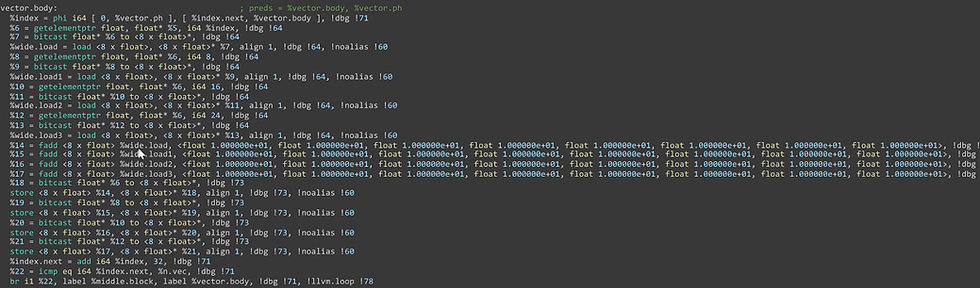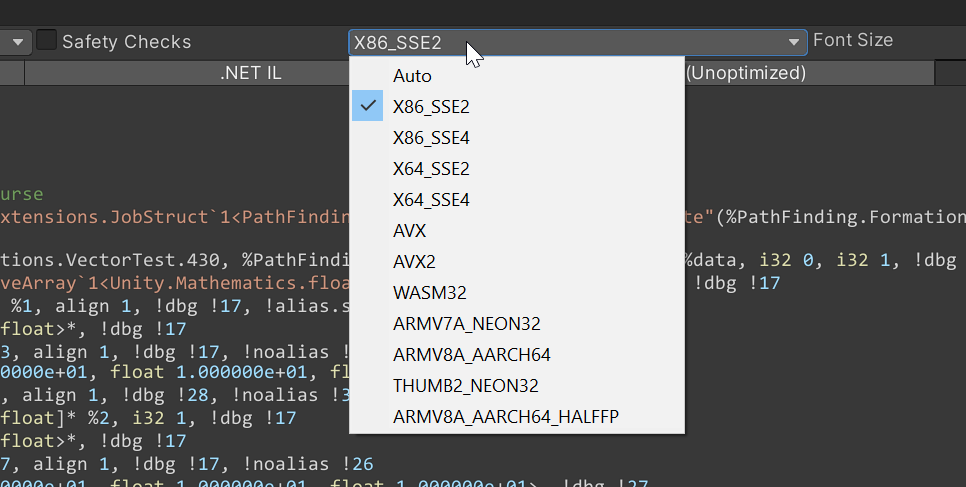top of page# Working with Burst

Updated: Nov 29, 2022

This is going to be a short blog and also incredibly technical compared to my other ones.

This time, I will share my experience with AVX in Burst. It might be super helpful to devs.

How does it all work in the Burst world? Vectorizing a simple loop

That should be simple right?

Well, apparently not.

Let’s take a look at a simple example :

[BurstCompile] public struct VectorTest: IJob { public int size; public NativeArray<float> data; public void Execute() { for (int i = 0; i < size; i++) { data[i] += 10; } } }

Here is the code it emits. The vectorized code is at the mouse pointer.

8 x float meaning 8 wide as expected from AVX 256.Another example is with Sse or streaming SIMD extensions. SIMD meaning single instruction with multiple data. As expected 128 bits wide, so 4xLet’s try changing to half data type. As half is well half of float, that means it should get 2x as wide or 32 right?Hmm

As you can see from the pictures there is no 32. What happened?

Well as you see avx2 nor SSE or anything, supports any halfs. They support shorts. Or bytes. Or doubles. What it does right now is convert the half to float then add it the slow non vectorized way.

Anyway, let's move to another example.

[BurstCompile] public struct VectorTest : IJob { public int size; public NativeArray<float3> data; public void Execute() { for (int i = 0; i < size; i++) { data[i] *= 10; } } }

I only changed float to float3, should be fine right? I mean after all data wise this is exactly the same as if I made data to be 3x as large.Oh no. What did we do wrong? Why is it not working? Let’s try random stuff. Imma skips to the chase and tell you what works.

[BurstCompile] public struct VectorTest : IJob { public int size; public NativeArray<float3> data; public void Execute() { for (int i = 0; i < 8; i++) { data[i] *= 10; } } }

What changed? Well I just replaced size with 8. Nothing too different right?

It does technically say it is vectorized right now, but going into the emitted IR, we see that no AVX instructions are used. We can verify that by switching to sse and see that no instructions changehere is the final emitted in reality:As we see, what it did was use SSE instructions.

Here is the assembly that is generatedAs we can see it contains the instruction vaddps

Which you can find at

And see it is a vector extension.

But really what is going on here?

Well, when you use float2/3/4 the compiler generates vector instructions individually.

[BurstCompile] public struct VectorTest : IJob { public int size; public NativeArray<float3> data; public quaternion rotation; public void Execute() { for (int i = 0; i < 1; i++) { var innerData = data[i]; for (int j = 0; j < 3; j++) { innerData[i]+=10; } } } }

So it vectorizes the inner loop and says done. And for the outer loop, well when the outer loop gets too large, it cannot unroll it.

Because unrolling is just copy-pasting the same code. But with different indexes. Which benefits, performance but we cannot do it endlessly. As the cost of loading new instructions become greater than what we save from avoiding looping.

Anyway if you need to vectorize that too, then just make the inner loop and outer loop like this.

Here is an example of me looping through units in a vectorized fashion

var indexOffset = 0; for (var i = 0; i < (unitCount + vectorSize - 1) / vectorSize; i++) { //this loop is vectorized for (var index = 0; index < vectorSize; index++) { var realIndex = index + indexOffset; var slot = freeSlots[realIndex]; var result = slot * spacing; result = math.mul(rotation, result); result += futureSight; result += formationCenter; tempMem[index] = result; } for (int index = 0; index < vectorSize; index++) { var result = tempMem[index]; var realIndex = index + indexOffset; if (realIndex >= unitCount) break; var unit = units[realIndex]; formationOffsets[unit.id] = result; Assert.IsTrue(math.isfinite(formationOffsets[unit.id]).All()); } indexOffset += 8; }

As you can see, in the vectorized loop I perform an expensive computation, mainly the quaternion with float3 multiplication. Which gets decomposed into roughly(not the same example)

As you can see it spams float 3 multiplications, honestly you can do a better job than it.Some manual vectorization

I wrote this code before figuring out, how to tame the Unity auto-vectorizer, so it was all manual.

What it does is simply calculate the distance between points in AVX way.

What we are trying to accomplish is implementing these functions

public static float dot(float2 x, float2 y) { return x.x * y.x + x.y * y.y; }

public static float lengthsq(float2 x) { return dot(x, x); }

public static float distancesq(float2 x, float2 y) { return lengthsq(y - x); }

var cellUnitPosVector = X86.Avx.mm256_load_ps(cellPositionsPtr + unitInCellIndex); //contains 4 unit positions //vectorPosition is just a vector 2 that is copy pasted 4 times var offsetCellPositions = X86.Avx.mm256_sub_ps(cellUnitPosVector, vectorPosition); //cellUnitPosVector - vectorPosition var dotMul = X86.Avx.mm256_mul_ps(offsetCellPositions, offsetCellPositions); //x.x * y.x and x.y * y.y, we can abuse the fact that both x and y are the same // x1 y1 x2 y2... // * // x1 y1 x2 y2... var dotAdd = X86.Avx.mm256_hadd_ps(dotMul, dotMul); //This results in a vector where each second value is the same as the previous. We need to convert it to v128 by discarding every second result if (sqrtDistance) { dotAdd = X86.Avx.mm256_sqrt_ps(dotAdd); } result = new float4 {  = dotAdd.Float0,  = dotAdd.Float1,  = dotAdd.Float4,  = dotAdd.Float5 };

As you can see, all the avx goodies are contained in the x86.Avx namespace.

We basically load 4 times the same float2. Eg: [1,2,1,2,1,2,1,2]

Do the y - x, with the mm256_sub_ps

Then in the dot function we need to basically do:

x.x * x.x , x.y * x.y

However we also need to do the + part.

So what we need is to sum every pair, and the result would be 4 float wide.

Looking at the intel website we see this instruction mm256_hadd_ps.Just divide the values in the braces by 32

As you can see, it adds into

dst = a + a

dst = a + a

...

dst = a + a

dst = a + a

And we manually extract all the values. Fortunately, that is cheap because the heavy lifting is done by the AVX instructions.

Before the end, we also check if we need to square root them. And it might be somewhat inefficient, since we only need to square root 4 out of 8, but doing it that way, it might require more work to actually be done, to prepare the data.